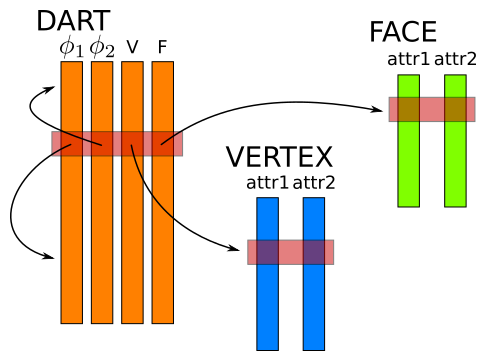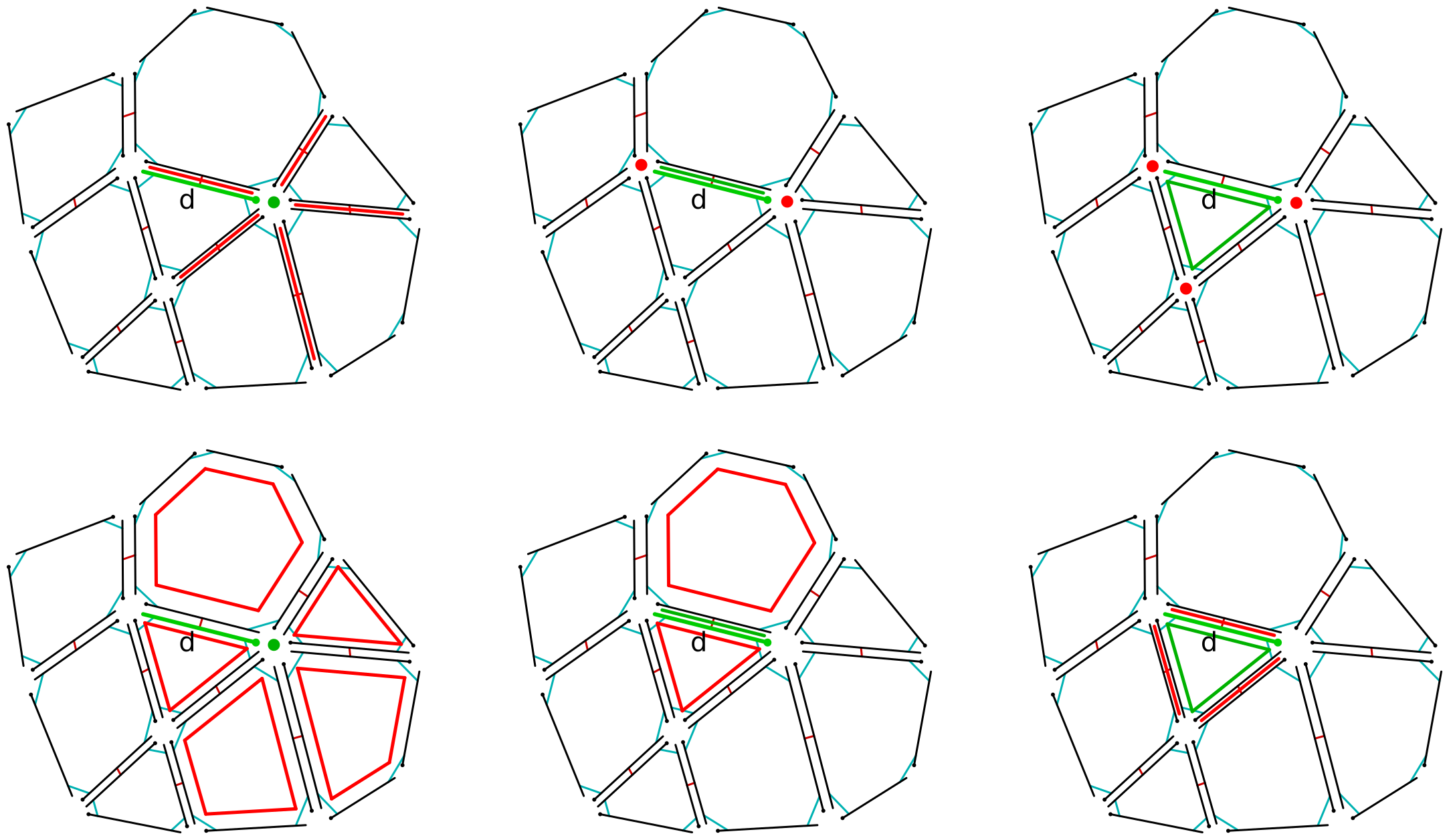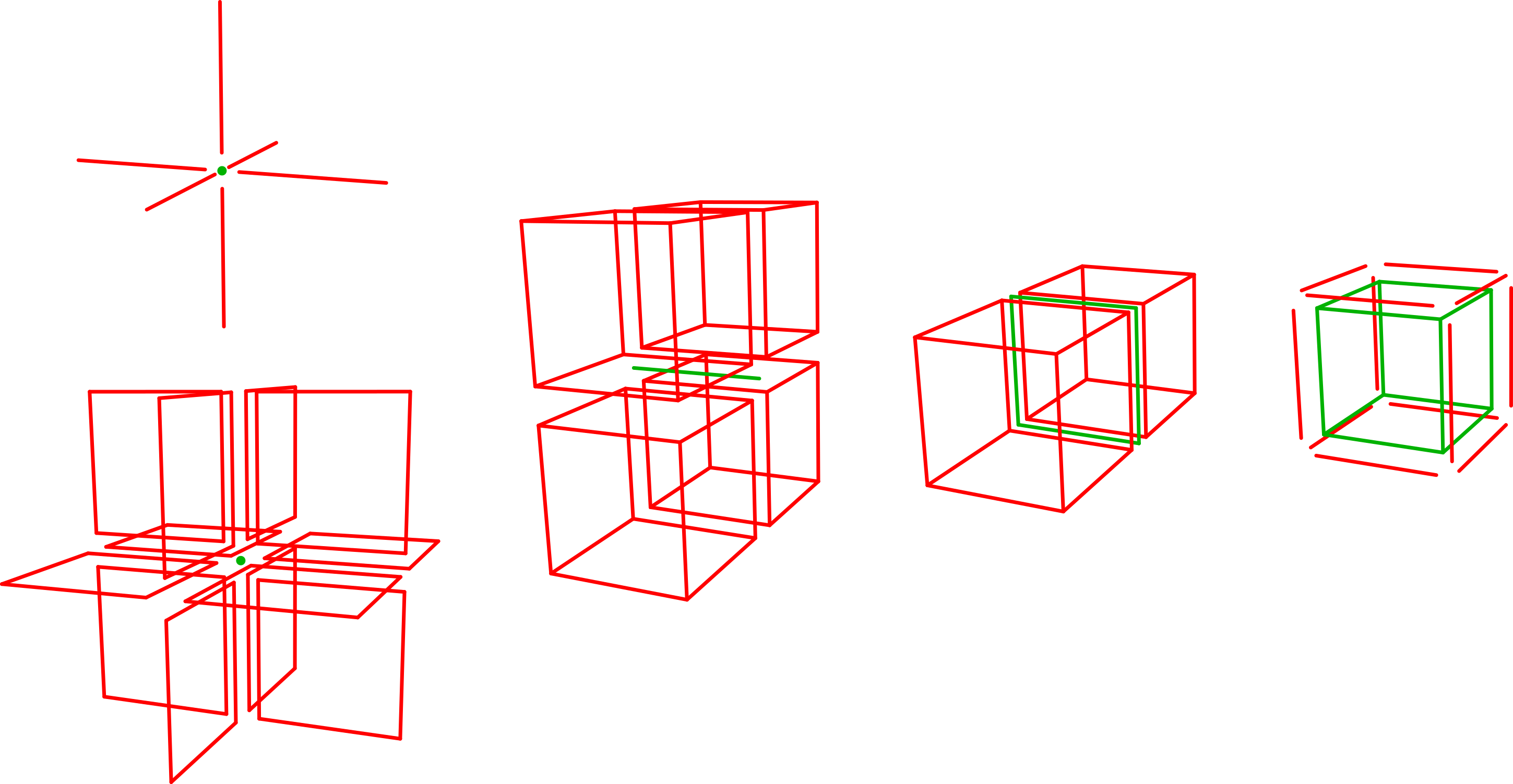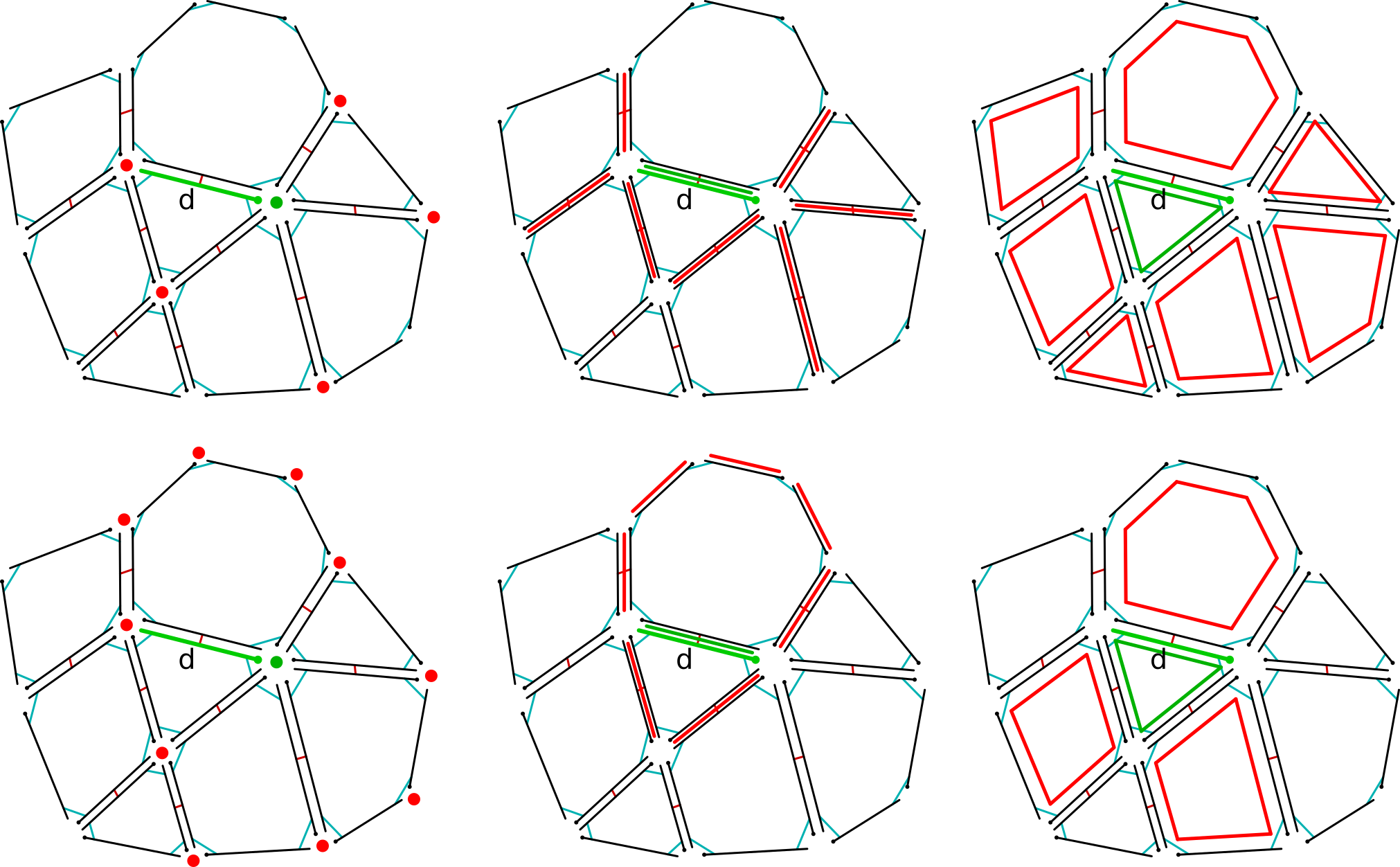View project on GitHub

# CGoGN

## A C++ library for the representation of meshes with Combinatorial Maps

CGoGN provides an efficient C++ implementation of oriented combinatorial maps. Its main features are combinatorial maps representation, manipulation, traversal and a dynamic attribute mechanism. It is also designed to take advantage of parallel architectures.

# Attribute containers

The central data structure used in CGoGN is attribute containers. An attribute container is a set of attributes of arbitrary type that all have the same number of records. Attributes can be dynamically added and removed in an attribute container. An index identifies a tuple of records, one in each attribute of the container. Records can be dynamically added and removed in an attribute container. Removed entries are actually only marked as removed and skipped during the traversals. They are left available for further record additions.

Each attribute is stored as a chunk array, i.e. a set of fixed size arrays. It allows the size of the attribute to grow while leaving all existing elements in place. Chunk size is chosen as a power of 2 so that the arithmetic operations needed to access the record of a given index (division and modulo) are very efficient.

A map in CGoGN is encoded as a set of attribute containers. The only mandatory container is the Dart container. According to the dimension of the map, it stores a variable number of attributes that encode the topological relations φ1, …, φn. Each dart being represented by its index in the Dart container, these attributes store indices of linked darts in the Dart container. For each embedded cell dimension, the Dart container maintains an attribute that stores for each dart the index of its corresponding cell orbit. These indices correspond to entries in a dedicated attribute container. For example, in the map illustrated in Figure 5, Vertex and Face cells are embedded. The Dart container maintains V and F attributes that store the Vertex and Face index of each dart. Obviously, as explained in the embedding section, all the darts of a same orbit share the same index. The Vertex and Face attribute containers store the data associated with each vertex and face cell of the map.Figure 5: Containers of a 2-dimensional combinatorial map with vertex and face embedding. The dart container stores φ1 and φ2 indices of linked darts and V and F indices of the associated vertices and faces attributes.

# Basic types

CGoGN defines symbols in the `cgogn` namespace which will be omitted in the following.

The most basic type defined in CGoGN is `Dart` which stores an index in the Dart container.

An enumeration of orbits is also defined. These orbits correspond to the different cells that can be defined in a combinatorial map. They are defined as follows:

``````enum Orbit: uint32
{
DART = 0,      // 1d vertex
PHI1,          // 1d cycle, 2d face
PHI2,          // 2d edge
PHI21,         // 2d vertex
PHI1_PHI2,     // 2d connected component, 3d volume
PHI1_PHI3,     // 3d face
PHI2_PHI3,     // 3d edge
PHI21_PHI31,   // 3d vertex
PHI1_PHI2_PHI3 // 3d connected component
};
``````

The `Cell<Orbit>` template class stores a Dart that is publicly available as a `dart` property. These cell types can actually be seen as orbit-typed Darts.

A combinatorial map is composed of a Dart container and a container for each of the orbits defined in the above enumeration. These containers can be used to store the attributes associated to the cells of each embedded orbit. Containers corresponding to non-embedded orbits will remain unused during the lifetime of the map.

Several map types are defined (one for each dimension). For example, a 2-dimensional combinatorial map can be declared like that: `CMap2 map;`.

Each map type provides several convenience internal definitions for its cells types. For example a `CMap2` provides the following cell types: `CMap2::Vertex`, `CMap2::Edge`, `CMap2::Face`, `CMap2::Volume`. These cells are actually defined respectively as: `Cell<Orbit::PHI21>`, `Cell<Orbit::PHI2>`, `Cell<Orbit::PHI1>`, `Cell<Orbit::PHI1_PHI2>` which correspond to the orbits of these cells in a 2-dimensional map.

A `CMap2::Vertex v` contains a Dart and can be thought of as the vertex of `v.dart`. Any other Dart of the same vertex orbit could equally represent the same cell.

# Attributes

Attributes of any type can be added in a map. The `Attribute<T, Orbit>` template class provides a way to access to the type `T` values associated to the `Cell<Orbit>` cells of a map. Here again, convenience definitions are provided in the map types: `CMap2::VertexAttribute<T>`, `CMap2::EdgeAttribute<T>`, etc.

For example, a Vertex attribute storing an integer value on each vertex can be added in a 2-dimensional map like that:

``````CMap2 map;
``````

An existing attribute can also be obtained by its name. As the requested attribute may not exist in the map, the validity of the obtained object can then be verified:

``````CMap2::FaceAttribute<double> area = map.get_attribute<double, CMap2::Face>("area");
if (!area.is_valid())
{
// there were no Face attribute of this type having this name in the map
}
``````

Attribute values can be traversed globally using the range-based for loop syntax:

``````for (const T& v : attr)
{
// do something with v
}
``````

Given a cell of the map, the value associated to this cell for an attribute can be directly accessed using the bracket operator:

``````// given two CMap2::Face f1, f2
area[f1] = 3.4;
double sum = area[f1] + area[f2];
``````

Under the hood, this operator will first query the embedding index of the given cell and then access to the value stored at this index in the corresponding Cell attribute container. The embedding index of a cell does not depend on the accessed attribute. As the bracket operator can also be given directly the index of the cell, in the case of accessing to multiple attributes for a same cell, it can be more efficient to first get the embedding index, and then directly use this index to access to the different values associated to the cell:

``````// given a CMap2::Face f
uint32 findex = map.embedding(f);
attr1[findex] = attr2[findex] + attr3[findex];
``````

Attributes can also easily be removed from a map:

``````map.remove_attribute(attr);
``````

# Global traversals

The cells of the map can be traversed using the `foreach_cell` method. This method takes a callable that expects a `Cell<Orbit>` as parameter. It is the type of this parameter that determines the type of the cells that will be traversed by the foreach method. For example, the following code will traverse the vertices of the map and call the given lambda expression on each vertex:

``````// given a CMap2 map
map.foreach_cell([&] (CMap2::Vertex v)
{
// do something with v
});
``````

No assumption can be made here about the `Dart` that represents each cell (e.g. `v.dart` in the previous example) when the processing function is called. Any dart of the same orbit could equally represent the same cell.

## Early stop

In order to stop the traversal before all the cells have been processed, the provided callable can return a boolean value. As soon as the callable returns false, the traversal is stopped. In the following example, we are looking for the first encountered vertex for which the Vec3 position value is on the negative side of the x=0 plan. If after the traversal, the declared Vertex is not valid, it means such a vertex has not been found in the map:

``````// given a CMap2 map
// given a CMap2::VertexAttribute<Vec3> position
CMap2::Vertex looked_up_v;
map.foreach_cell([&] (CMap2::Vertex v) -> bool
{
if (position[v] < 0.0)
{
looked_up_v = v;
return false;
}
return true;
});
if (!looked_up_v.is_valid())
{
// no such vertex was found
}
``````

## Parallelism

CGoGN can take advantage of parallel architectures to speed-up global traversals. A parallel traversal of the cells can be done using the `parallel_foreach_cell` method. The processing of the cells of the map will be spread among a number of threads that depends on the detected underlying hardware concurrency. In the following example, the displacement value value of each vertex is added to its position:

``````// given a CMap2 map
// given two CMap2::VertexAttribute<Vec3> position, displacement
map.parallel_foreach_cell([&] (CMap2::Vertex v)
{
position[v] += displacement[v];
});
``````

It is also possible to aggregate results coming from the parallel processing of several threads. In the following example, the average value of an Edge attribute is computed in parallel. Each thread accumulates the values and number of elements corresponding to the fraction of the mesh that it processes, and then the global result is computed:

``````// given a CMap2 map
// given a CMap2::EdgeAttribute<double> length

map.parallel_foreach_cell([&] (CMap2::Edge e)
{
});

double sum = 0.0;
for (double d : sum_per_thread) sum += d;
uint32 nb = 0;
for (uint32 n : nb_per_thread) nb += n;

double average = sum / double(nb);
``````

Note that early stop is not available when doing parallel traversals.

In their simplest form, the `foreach_cell` and the `parallel_foreach_cell` methods process all the cells of the map. A second parameter, which we call a Mask can be given to these methods and alter the way they work.

### Filtering functions

The most simple Mask takes the form of a callable, that takes as parameter the same type of Cell than the processing callable. This filtering callable returns a boolean value, and for each cell of the map, the processing callable will only be called if the filtering callable evaluated to true.

In the following example, the function will print only the faces for which the area is above a given threshold:

``````void print_faces(const CMap2& map, const CMap2::FaceAttribute<double>& area, double threshold)
{
map.foreach_cell(
[] (CMap2::Face f) { std::cout << f << std::endl; },
[&] (CMap2::Face f) -> bool { return area[f] > threshold; }
);
}
``````

Of course, things become much more interesting and reusable when the function takes the map and a Mask as parameters, and can focus on what it does without even knowing how the given Mask is altering the traversal:

``````template <typename MASK>
{
map.foreach_cell(
[] (CMap2::Face f) { std::cout << f << std::endl; },
);
}
``````

The filtering Mask can then be defined directly when calling the function, using any local data to do its work:

``````// given a CMap2 map
// given a CMap2::FaceAttribute<double> area
// given a double value threshold
print_faces(map, [&] (CMap2::Face f) -> bool { return area[f] > threshold; });
``````

### Cell filters

There are cases in which a function has to traverse several types of cells. Passing an additional Mask parameter for each type of cell would not be a very versatile solution. That is why the `foreach_cell` and `parallel_foreach_cell` methods can also accept an instance of the `CellFilters` class as their second parameter.

Such an object can store a filtering function for each type of cell. In the following example, the `CellFilters` instance is configured to keep the vertices for which a boolean attribute is true and the edges for which the attribute is true for its two incident vertices:

``````// given a CMap2 map
// given a CMap2::VertexAttribute<bool> selected

CellFilters cf;
cf.set_filter<CMap2::Vertex>([&] (CMap2::Vertex v) { return selected[v]; });
cf.set_filter<CMap2::Edge>([&] (CMap2::Edge e)
{
return
selected[CMap2::Vertex(e.dart)] &&
selected[CMap2::Vertex(map.phi2(e.dart))];
});

// this function needs to traverse vertices and edges
{
map.parallel_foreach_cell(
[] (CMap2::Vertex v) { /* do something with v */ },
);
map.parallel_foreach_cell(
[] (CMap2::Edge e) { /* do something with e */ },
);
}

f(map, cf);
``````

If a `CellFilters` object is used to traverse a type of cell for which no filter has been configured, the `foreach_cell` method prints a warning.

### Cell traversors

Filtering functions are a great and versatile way to customize cell traversals. They are particularly well adapted to highly variable settings where the set of filtered cells changes regularly w.r.t. the filtering function. However, under the hood, the complete map is still traversed using classical algorithms that enumerate all the darts and use markers (a boolean attribute) to tag the darts of the processed orbits.

Other types of objects, derived from the `CellTraversor` class, can be used as a Mask and given as a second parameter to the `foreach_cell` or `parallel_foreach_cell` method. Any `CellTraversor` should provide a `begin<CellType>` and `end<CellType>` template methods that return an internal `const_iterator` type instance. These methods are then used by the `foreach_cell` or `parallel_foreach_cell` method to completely overload the classical traversal. Several `CellTraversors` are already provided in CGoGN.

QuickTraversor

The goal of a `QuickTraversor` is to avoid the enumeration of all the darts and the marking/unmarking cost related to the usage of a marker. To achieve this, it creates and maintains an Attribute of type `Dart` in the attribute container of the traversed cell type. Calling the `build<CellType>` method will fill this attribute with one representing dart per cell. When the `QuickTraversor` is given to the `foreach_cell` or `parallel_foreach_cell` method, its internal attribute is used to directly enumerate the cells without having to manage any marker, providing a significative speed-up.

However, in order to keep the `QuickTraversor` in sync with the map, special care must be taken when the connectivity is modified:

• when a new orbit is created, one of its darts has to be written as representing dart in the internal attribute. This can be done by passing the new cell to the `update` method.
• when an existing orbit is modified, if one or more darts have been removed, the representing dart stored in the internal attribute may have been removed. A valid dart can be chosen again by passing the cell to the `update` method.
• when an orbit is completely deleted, nothing has to be done as the attributes of this cell will not be traversed anymore.

In the following example, a `QuickTraversor` is built for the vertices of the map. Then an edge is cut, inserting a new vertex. In order for this new vertex to have a valid representing dart in the `QuickTraversor` internal attribute, this new vertex is updated:

``````// given a CMap2 map
CMap2::QuickTraversor qtrav(map);
qtrav.build<CMap2::Vertex>();
map.foreach_cell(
[&] (CMap2::Vertex v) { /* do something with v */ },
qtrav
);
// given a CMap2::Edge e
CMap2::Vertex v = map.cut_edge(e);
qtrav.update(v);
``````

The selection of the dart that represents each cell can be customized by giving an additional function as parameter to the `build` and `update` methods. This function should take a `Cell` as parameter and return a `Dart`. This customization is useful if you want to be able to make assumptions about the dart that represents each cell when it is given to the processing function during a traversal.

FilteredQuickTraversor

A `FilteredQuickTraversor` is a variation of a `QuickTraversor`. In this modified version, the internal attribute is still filled with one dart per cell when calling the `build` method and the same updates have to be done in order to keep it in sync with the map. The difference is an additional `set_filter<CellType>` method that provides a filter which is evaluated on-the-fly each time the `FilteredQuickTraversor` is used for the traversal of the cells. It can be seen as a combination of the efficiency of the marker-less traversal and the versatility of the on-the-fly cells filtering.

The dart selection customization is also available by giving a dart selection function as an additional parameter to the `build` and `update` methods.

CellCache

With the previous Masks, even when using a filtering function, all the cells of the map are always traversed and the filtering function is evaluated on-the-fly for each cell in order to decide if the processing function should be called on it or not. This can be great in a dynamic environment where the set of filtered cells changes regularly. But if the set of filtered cells is stable, and moreover if this set is small w.r.t. the number of cells of the map, a more efficient solution can be proposed.

A `CellCache` can store a set of cells for each type of cell. When a `CellCache` is given as a second argument to the `foreach_cell` or `parallel_foreach_cell` method, its sets will be directly traversed.

Its main public method is `build<CellType>` which builds the set of the mentioned cell type. If no argument is given, all the cells of the map will be put into the cache. However, the build method can also take a Mask as an optional argument to restrict the cached cells to the ones that are visible with the given Mask. Any kind of supported Mask, i.e. a filtering function, a `CellFilter` or a `CellTraversor` can be used. Of course, you just cannot give the one that you are trying to build..

In the following example, all the degree 5 vertices are put into a cache which is then used in a traversal that is efficient and restricted to those vertices:

``````// given a CMap2 map
CMap2::CellCache cache(map);
cache.build<CMap2::Vertex>([&] (CMap2::Vertex v)
{
return map.degree(v) == 5;
});
map.foreach_cell(
[&] (CMap2::Vertex v) { /* do something with v */ },
cache
);
``````

Note that if a new cell that satisifies the filter given to the build method is inserted into the map, it will not be part of a cache that has already been built.

Note also that if any cell stored in the cache is deleted from the map, the cache is invalid and any subsequent traversal with this cache will fail.

The “snapshot” property of the `CellCache` can be particularly exploited within algorithms that insert new cells during the traversal of the map. Indeed, without such a mechanism, the newly inserted cells could also be traversed resulting in a possible infinite loop. In the following example, all the edges of the map are cut by inserting a new vertex. Only the edges that existed at the moment the cache was built are considered:

``````// given a CMap2 map
CMap2::CellCache cache(map);
cache.build<CMap2::Edge>();
map.foreach_cell([&] (CMap2::Edge e) { map.cut_edge(e); }, cache );
``````

## Local traversals

All the incidence and adjacency relations between cells are encoded within a combinatorial map. We first give an intuitive definition of what we mean by incidence and adjacency relations and then show how to make these neighborhood traversals in CGoGN.

### Incidence

Let C1 and C2 be two cells of different dimensions with dim(C1) > dim(C2). C2 is said to be incident to C1 if it belongs to the sub-tree under C1 in the incidence graph (for example, the four vertices and the four edges that bound a quad face in a 2D mesh are said to be incident to this face). C1 is said to be incident to C2 if it is part of the ancestors of C2 in the incidence graph (for example, the six edges and the six faces around a vertex in a regular 2D triangle mesh are said to be incident to this vertex).

The following Figure 6 illustrates the incident cells in a 2-dimensional map and Figure 7 illustrates some of the incident cells in a 3-dimensional map.Figure 6: Incident cells traversals in a 2-dimensional map. Central cell is drawn in green and incident cells are drawn in red. Top-left: incident edges of a vertex; Bottom-left: incident faces of a vertex; Top-middle: incident vertices of an edge; Bottom-middle: incident faces of an edge; Top-right: incident vertices of a face; Bottom-right: incident edges of a face.Figure 7: Some of the incident cells traversals in a 3-dimensional map. Central cell is drawn in green and incident cells are drawn in red. From left to right: incident edges of a vertex and incident faces of a vertex; incident volumes of an edge; incident volumes of a face; incident edges of a volume.

Two cells of equal dimension C1 and C2 are said to be adjacent if they share a common incident cell. For example: two vertices are said to be adjacent through an edge if they are both incident to a same edge; two faces are said to be adjacent through a vertex if they are both incident to a same vertex.Figure 8: Adjacent cells traversals in a 2-dimensional map. Central cell is drawn in green and adjacent cells are drawn in red. Top-left: adjacent vertices through an edge; Bottom-left: adjacent vertices through a face; Top-middle: adjacent edges through a vertex; Bottom-middle: adjacent edges through a face; Top-right: adjacent faces through a vertex; Bottom-right: adjacent faces through an edge.

### Local traversal methods

CGoGN provides methods to traverse all these local neighborhoods. These methods all work in the same way: the callable given as second parameter is called on all the requested incident or adjacent cells of the cell given as first parameter. If this callable returns a boolean value, the traversal is stopped as soon as this value is false.

For example, in a 2-dimensional map, the local neighborhood traversal functions are the following:

• `foreach_incident_edge(CMap2::Vertex, [] (CMap2::Edge) {})`
• `foreach_incident_face(CMap2::Vertex, [] (CMap2::Face) {})`
• `foreach_adjacent_vertex_through_edge(CMap2::Vertex, [] (CMap2::Vertex) {})`
• `foreach_adjacent_vertex_through_face(CMap2::Vertex, [] (CMap2::Vertex) {})`
• `foreach_incident_vertex(CMap2::Edge, [] (CMap2::Vertex) {})`
• `foreach_incident_face(CMap2::Edge, [] (CMap2::Face) {})`
• `foreach_adjacent_edge_through_vertex(CMap2::Edge, [] (CMap2::Edge) {})`
• `foreach_adjacent_edge_through_face(CMap2::Edge, [] (CMap2::Edge) {})`
• `foreach_incident_vertex(CMap2::Face, [] (CMap2::Vertex) {})`
• `foreach_incident_edge(CMap2::Face, [] (CMap2::Edge) {})`
• `foreach_adjacent_face_through_vertex(CMap2::Face, [] (CMap2::Face) {})`
• `foreach_adjacent_face_through_edge(CMap2::Face, [] (CMap2::Face) {})`
• `foreach_incident_vertex(CMap2::Volume, [] (CMap2::Vertex) {})`
• `foreach_incident_edge(CMap2::Volume, [] (CMap2::Edge) {})`
• `foreach_incident_face(CMap2::Volume, [] (CMap2::Face) {})`

The following example illustrates a function that computes the average of vertex attribute values over the vertices incident to a face in a 2-dimensional map:

``````template <typename T>
T average(const CMap2& map, CMap2::Face f, const CMap2::VertexAttribute<T>& attribute)
{
T result(0);
uint32 nbv = 0;
map.foreach_incident_vertex(f, [&] (CMap2::Vertex v)
{
result += attribute[v];
++nbv;
});
return result / nbv;
}
``````

Given that all the equivalent neighborhood traversal functions are also defined in 3-dimensional maps, and that the incident vertices can be traversed for any cell of dimension k > 0, the previous function can be easily generalized to the computation of the average of vertex attribute values over the vertices incident to a k-cell in a n-dimensional map:

``````template <typename T, typename CellType, typename MAP>
T average(const MAP& map, CellType c, const typename MAP::template VertexAttribute<T>& attribute)
{
T result(0);
uint32 nbv = 0;
map.foreach_incident_vertex(c, [&] (typename MAP::Vertex v)
{
result += attribute[v];
++nbv;
});
return result / nbv;
}
``````

# Properties

Several methods can provide some properties about the map or the cells.

• nb_cells
• nb_boundaries
• same_cell
• degree
• codegree
• is_incident_to_boundary